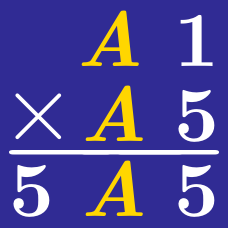Logic

# Cryptogram Warmup

$\Large \begin{array} {c c c } & 3 & \color{#EC7300}{X} \\ + & & 7 \\ \hline & 4 & 1 \\ \end{array}$

What digit in place of $\color{#EC7300}{X}$ would make the above summation true?

$\Large \begin{array} {c c c } & 2 & \color{#3D99F6}{A} \\ \times & & 4 \\ \hline & 9 & 6 \\ \end{array}$

What digit in place of $\color{#3D99F6}{A}$ would make this multiplication true?

$\Large \begin{array} {c c c } & 1 & \color{#3D99F6}{E} \\ \times & & \color{#3D99F6}{E} \\ \hline & 9 & \color{#3D99F6}{E} \\ \end{array}$

What digit in place of $\color{#3D99F6}{E}$ would make this multiplication true?

$\Large \begin{array} {c c c } & & \color{#20A900}{C} \\ + & \color{#20A900}{C} & \color{#20A900}{C} \\ \hline & \color{#69047E}{D} & 4 \\ \end{array}$

In this cryptogram, $\color{#20A900}{C}$ and $\color{#69047E}{D}$ represent two different digits. What is the value of $\color{#20A900}{C}$?

$\Large \begin{array} { c c c } & 1 & \color{#EC7300}{B} \\ + & \color{#EC7300}{B} & 6 \\ \hline & 7 & 1 \\ \end{array}$

What digit in place of $\color{#EC7300}{B}$ would make this sum true?

×